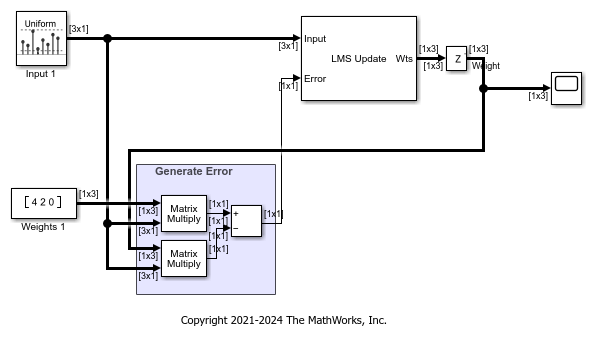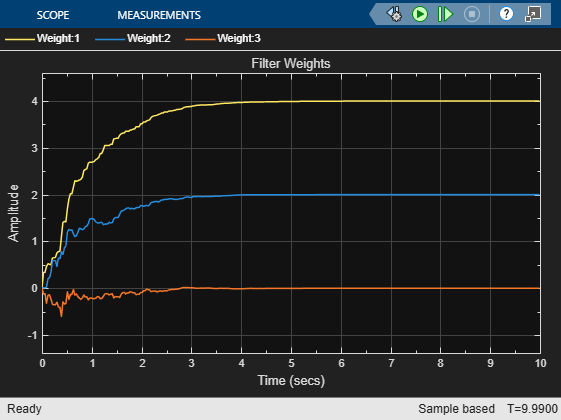# Model Adaptive Linear Combiner using LMS Update Block

This example shows to use the LMS Update block as an adaptive linear combiner.

The adaptive linear combiner (ALC) resembles the adaptive tapped delay-line FIR filter except that there is no relationship between the input elements. When the LMS Update block is set to operate as an adaptive linear combiner, the block expects the input elements to be in the form of a vector. The block does not buffer the input samples in this case.

Open the model. The LMS Update block in the model has the following settings:

• Number of adaptive filters is set to 1.

• Filter length is set to 3 taps.

• Adaptive filter mode is set to `Adaptive linear combiner`.

The signal at the Input port is a random signal with a uniform distribution. The input signal has a sample time of 0.01 seconds and contains 3 sample per frame.

The error signal is the difference between the desired signal and the actual output. The first Matrix Multiply block multiplies the input vector with the actual filter weights to generate the desired signal. The second Matrix Multiply block multiplies the input vector with the weights estimated by the LMS Update block to generated the acutal output. The difference between the desired signal and the actual output is the error signal which is input back to LMS Update block through the Error port. This error signal is used to further adapt the filter in the direction of convergence.

Run the model. The weights estimated by the LMS Update block for both the filters converge towards the actual weights.